# Class 11 Maths NCERT Solutions for Chapter 8 Binomial Theorem Exercise 8.1### Binomial Theorem Exercise 8.1 Solutions

1. Expand the expression (1– 2x)5

Solution

By using Binomial Theorem, the expression (1-2x) can be expanded as
(1 -2x)5
= 5C0 (1)55C1 (1)4 (2x) + 5C2 (1)3 (2x)2 - 5C3 (1)2 (2x)3 + 5C4 (1)1(2x)4 + 5C5 (2x)5
= 1 – 5(2x) + 10(4x2 ) – 10(8x3 )+ 5(16x4 ) – (32x5 )
= 1 – 10x + 40x2 – 80x3 + 80x4 – 32x5

2. Expand the expression (2/x - x/2)5

Solution

By using Binomial Theorem, the expression (2/x - x/2)5 can be expanded as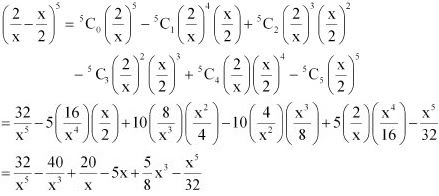3. Expand the expression (2x – 3)6 .

Solution

By using Binomial theorem, the expression (2x - 3)6 can be expanded as
(2x - 3)6
= 6C0 (2x)66C1 (2x)5 (3) + 6C2 (2x)4 (3)26C3 (2x)3 (3)3 + 6C4 (2x)2 (3)46C5 (2x) (3)5 + 6C6 (3)6
= 64x6 – 6(32x5 )(3) + 15(16x4 )(9) – 20(8x3 )(27) + 15(4x2) (81) – 6(2x)(243) + 729
= 64x6 – 576x5 + 2160x4 – 4320x3 + 4860x2 – 2916x + 729

4. Expand the expression (x/3 + 1/x)5

Solution

By using Binomial Theorem, the expression (x/3 + 1/x)5 can be expanded as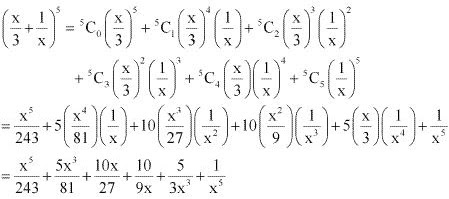5. Expand (x + 1/x)6

Solution

By using Binomial Theorem, the expression(x + 1/x)6 can be expanded as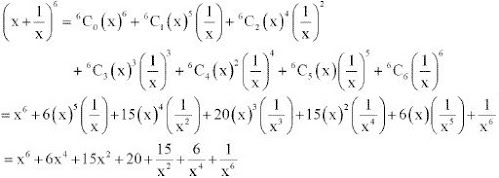6. Using Binomial Theorem, evaluate (96)3

Solution

96 can be expressed as the sum or difference of two numbers whose powers are easier to calculate and then, binomial theorem can be applied.
It can be written that, 96 = 100 - 4
∴ (96)3 = (100 - 4)3
3C0 (100)3 - 3C1 (100)2 (4) + 3C2 (100)(4)2 - 3C3 (4)3
= (100)3 - 3(100)2 (4) + 3(100)(4)2 - (4)3
= 1000000 - 120000 + 4800 - 64
= 884736

7. Using Binomial Theorem, evaluate (102)5

Solution

102 can be expressed as the sum or difference of two numbers whose powers are easier to calculate and then, Binomial Theorem can be applied.
It can be written that, 102 = 100 + 2
∴ (102)5 = (100 + 2)5
5C0(100)5 - 5C1(100)4 (2) + 5C2(100)3 (2)2 - 5C3(100)2 (2)3 + 5C4(100)(2)4 + 5C5(2)5
= (100)5 + 5(100)4 (2) + 10(100)3 (2)2 + 10(100)2 (2)3 + 5(100)(2)4 +(2)5
= 10000000000 + 1000000000 + 40000000 + 800000 + 8000 + 32
= 11040808032

8. Using Binomial Theorem, evaluate (101)4

Solution

101 can be expressed as the sum or difference of two numbers whose powers are easier to calculate and then, Binomial Theorem can be applied.
It can be written that, 101 = 100 + 1
∴ (101)4  = (100 + 1)4
4C0(100)4 - 4C1(100)3 (1) + 4C2(100)2 (1)2 - 4C3(100)(1)3 + 4C4(1)4
= (100)4 + 4(100)3 + 6(100)2 + 4(100) + (1)4
= 100000000 +4000000 + 60000 + 400 + 1
= 104060401

9. Using Binomial Theorem, evaluate (99)5

Solution

99 can be written as the sum or difference of two numbers whose powers are easier to calculate and then, Binomial Theorem can be applied.
It can be written that, 99 = 100 – 1
∴ (99)5 = (100 - 1)5
5C0 (100)5 - 5C1 (100)4 (1) + 5C2 (100)3 (1)2 - 5C3 (100)2 (1)3 + 5C4 (100)(1)4 + 5C5 (1)5
= (100)5 - 5(100)4 + 10(100)3 - 10(100)2 + 5(100) - 1
= 10000000000 - 500000000 + 10000000 - 100000 + 500 - 1
= 10010000500 - 500100001
= 9509900499

10. Using Binomial Theorem, indicate which number is larger (1.1)10000 or 1000.

Solution

By splitting 1.1 and then applying Binomial Theorem, the first few terms of (1.1)10000 can be obtained as
(1.1)10000 = (1 + 0.1)10000
10000C0 - 10000C1 (1.1) + Other positive terms
= 1 + 10000 × 1.1 + Other positive terms
= 1 + 11000 + Other positive terms
> 1000
Hence, (1.1)10000 > 1000

11. Find (a + b)4 - (a - b)4. Hence, evaluate (√3 + √2)4 - (√3 - √2)4

Solution

Using Binomial Theorem, the expressions, (a + b)4 and (a - b)4 , can be expanded as
(a + b)4 = 4C0a4 + 4C1a3b + 4C2a2b2 + 4C3ab3 + 4C4b4
(a - b)4 =  4C0a4 - 4C1a3b + 4C2a2b2 - 4C3ab3 + 4C4b4
∴ (a + b)4 - (a - b)4 = 4C0a4 + 4C1a3b + 4C2a2b2 + 4C3ab3 + 4C4b4 - [4C0a4 - 4C1a3b + 4C2a2b2 - 4C3ab3 + 4C4b4]
= 2(4C1a3b + 4C3ab3) = 2(4a3b + 4ab3
= 8ab (a2 + b2 )
By putting a = √3 and b = √2 , we obtain
(√3 + √2)4 - (√3 - √2)4 = 8(√3)(√2) {(√3)2  + (√2)2 }
= 8(√6) (3 + 2) = 40√6

12. Find (x + 1)6 + (x -1)6. Hence or otherwise evaluate (√2 + 1)6 + (√2 - 1)6

Solution

Using Binomial Theorem, the expressions, (x + 1)6 and (x - 1)6 , can be expanded as
(x + 1)6  = 6C0 x6 + 6C1 x5  + 6C2 x4 + 6C3 x3 + 6C4 x2 + 6C5 x + 6C6
(x - 1)6 =  6C0 x6 - 6C1 x5  + 6C2 x4 - 6C3 x3 + 6C4 x2 - 6C5 x + 6C6
∴ (x + 1)6 + (x -1)6 = 2[6C0 x6 + 6C1 x5  + 6C2 x4 +  6C4 x2 + 6C6]
= 2[x6 + 15x4 + 15x2 + 1]
By putting x = √2, we obtain
(√2 + 1)6  + (√2 - 1)6  = 2[(√2)6 + 15(√2)4 + 15(√2)2 + 1 ]
= 2(8 + 15 × 4 + 15 × 2 + 1)
= 2(8 + 60 + 30 + 1)
= 2(99) = 198

13. Show that 9n+1 – 8n – 9 is divisible by 64, whenever n is a positive integer.

Solution

In order to show that 9n+1 - 8n - 9 is divisible by 64, it has to be proved that,
9n+1  - 8n - 9 = 64k, where k is some natural number.
By Binomial Theorem,
(1 + a)m =  mC0 + mC1 a + mC2 a2 + .... + mCm am
For a = 8 and m = n + 1, we obtain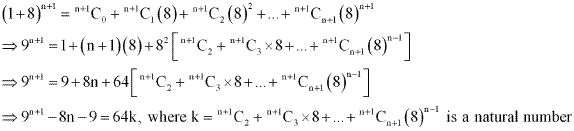Thus, 9n+1  - 8n - 9 is divisible by 64, whenever n is positive integer.

14. Prove that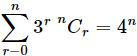Solution

By Binomial Theorem,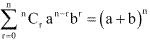By putting b = 3 and a = 1 in the above equation, we obtain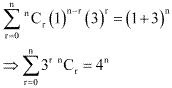Hence, proved.